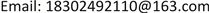﻿ 多个对数正态总体共同均值的估计问题研究 Research on the Estimation of Common Mean for Multiple Log-Normal Populations

Statistics and Application
Vol. 07  No. 05 ( 2018 ), Article ID: 27198 , 5 pages
10.12677/SA.2018.75060

Research on the Estimation of Common Mean for Multiple Log-Normal Populations

Qiuyue Wei1, Zeyu Li2, Weiyan Mu1

1School of Science, Beijing University of Civil Engineering and Architecture, Beijing

2Canvard College, Beijing Technology and Business University, BeijingReceived: Oct. 1st, 2018; accepted: Oct. 15th, 2018; published: Oct. 22nd, 2018ABSTRACT

If the random variable $X=lnY~N\left(\mu ,{\sigma }^{2}\right)$ , then the random variable $X$ follows the log-normal distribution, which is used to describe a class of positive right-skewed data and the practical application is very extensive  . In many cases, the source of data has different backgrounds, for a single population research  has been unable to meet our needs, so the main purpose of this time is to study their common parameters based on several populations. In this paper, the generalized pivot of the mean  is given for a single sample by means of generalized inference, and then the weighted average of the generalized pivot is given for different populations of common mean based on the sample size extracted from each population and the generalized pivot of approximate sample variance. Then the generalized confidence interval of the common mean is obtained. The probability of coverage is close to the confidence level using R.

Keywords:Log-Normal Distribution, Generalized Pivotal Quantity, Generalized Confidence Interval, Weighted Average, R1北京建筑大学理学院，北京

2北京工商大学嘉华学院，北京Copyright © 2018 by authors and Hans Publishers Inc.1. 引言

2. 广义枢轴量和广义置信区间

$f\left(y\right)=f\left(y\right)=\left\{\begin{array}{c}\frac{1}{\sqrt{2\pi }\sigma y}{\text{e}}^{-{\left(\mathrm{ln}y-\mu \right)}^{2}/2{\sigma }^{2}}\\ 0\end{array}\begin{array}{c}y>0\\ y\le 0\end{array}$

2.1. 广义枢轴量和广义置信区间

1) 对给定的 $x$$R\left(X;x,\eta \right)$ 的分布与未知参数 $\eta =\left(\theta ,\delta \right)$ 无关；

2) 观测值 $r=R\left(x;x,\eta \right)$ 与讨厌参数 $\delta$ 无关。

$P\left(R\left(x;x,\eta \right)\in {C}_{\gamma }\right)=\gamma$

${\Theta }_{\gamma }=\left\{\theta |R\left(x;x,\eta \right)\in {C}_{\gamma }\right\}$

$T+R=g\left(\theta \right)$ ，其中 $g\left(\theta \right)$ 为兴趣参数的函数，因此可以通过构造广义枢轴量的方法来进行假设检验，且其相应的广义 $p$ 值可以通过二者的关系计算得到。

2.2. Fiducial广义枢轴量

$R\left(X;x,\eta \right)$ 是关于X，x和 $\eta$ 的参数，其中 $\eta =\left(\theta ,\delta \right)$$\theta$ 是兴趣参数， $\delta$ 是讨厌参数，并且满足以下条件：

1) 对给定的 $x$$R\left(X;x,\eta \right)$ 的分布与未知参数 $\eta =\left(\theta ,\delta \right)$ 无关；

2) 观测值 $R\left(x,x,\eta \right)=\theta$

3. 提出的方法

3.1. 广义枢轴量的构造

${X}_{ij}=\mathrm{log}{Y}_{ij}~N\left({\mu }_{i},{\sigma }_{i}^{2}\right)$ ，因此我们有：

$\theta =\mathrm{exp}\left({\mu }_{i}+{\sigma }_{i}^{2}/2\right)$$i=1,2,\cdots ,k$

${\stackrel{¯}{X}}_{i}$${S}_{i}^{2}$ 分别表示从第 $i$ 个对数正态总体样本数据做对数转换后的均值与方差， ${X}_{ij}=\mathrm{log}{Y}_{ij}\left(j=1,2,\cdots ,{n}_{i}\right)$ ，且令 ${\stackrel{¯}{x}}_{i}$${s}_{i}^{2}$ 分别表示他们的观测值。由于：

$\frac{{\stackrel{¯}{X}}_{i}-{\mu }_{i}}{\sqrt{{\sigma }_{i}^{2}/n}}={Z}_{i}~N\left(0,1\right)$

$\left({n}_{i}-1\right){S}_{i}^{2}/{\sigma }_{i}^{2}={V}_{i}~{\chi }_{{n}_{i}-1}^{2}$

${R}_{{\sigma }_{i}^{2}}=\frac{\left({n}_{i}-1\right){s}_{i}^{2}}{{V}_{i}}$(1)

${R}_{{\mu }_{i}}={\stackrel{¯}{x}}_{i}-\frac{{Z}_{i}}{\sqrt{{U}_{i}}}\sqrt{\frac{\left({n}_{i}-1\right){s}_{i}^{2}}{{n}_{i}}}$ (2)

${\stackrel{^}{\theta }}^{\left(i\right)}$ 的样本方差可以近似为：

$\mathrm{var}\left({\stackrel{^}{\theta }}^{\left(i\right)}\right)={\sigma }_{i}^{2}\left(1+{\sigma }_{i}^{2}/2\right)\mathrm{exp}\left(2{\mu }_{i}+{\sigma }_{i}^{2}\right)$  (5)

${R}_{\theta }=\frac{{\sum }_{i=1}^{k}{R}_{{w}_{i}}{R}_{\theta }^{\left(i\right)}{n}_{i}}{{\sum }_{i=1}^{k}{R}_{{w}_{i}}{n}_{i}}$(6)

${R}_{\mathrm{var}\left({\stackrel{^}{\theta }}^{\left(i\right)}\right)}={R}_{{\sigma }_{i}^{2}}\left(1+{R}_{{\sigma }_{i}^{2}}/2\right)\mathrm{exp}\left(2{R}_{{\mu }_{i}}+{R}_{{\sigma }_{i}^{2}}\right)$ (8)

3.2. 算法

1) 计算 ${\stackrel{¯}{x}}_{i}$${s}_{i}^{2}$$i=1\cdots k$

2) 产生 ${V}_{i}~{\chi }_{{n}_{i}-1}^{2}$ 的实现值，然后按(1)给出的公式计算 ${R}_{{\sigma }_{i}^{2}}$$i=1\cdots k$

3) 产生 ${Z}_{i}~N\left(0,1\right)$${U}_{i}~{\chi }_{{n}_{i}-1}^{2}$ 的相互独立的实现值，然后根据(2)给出的公式计算 ${R}_{{\mu }_{i}}$$i=1\cdots k$

4) 根据公式(3)计算 ${R}_{\theta }^{\left(i\right)}$$i=1\cdots k$

5) 重复步骤2~3共 $t$ 次，根据公式(7)和(8)计算 ${R}_{{w}_{i}}$

6) 根据公式(6)计算得到 ${R}_{\theta }$

7) 重复步骤2~6共m次，得到一系列 ${R}_{\theta }$

8) 将这以系列 ${R}_{\theta }$ 案从小到大排列。

4. 模拟研究与结论Table 1. Empirical coverage probabilities of 90 percent two-sided confidence bounds for the common mean

Research on the Estimation of Common Mean for Multiple Log-Normal Populations[J]. 统计学与应用, 2018, 07(05): 516-520. https://doi.org/10.12677/SA.2018.75060

1. 1. 叶林, 邓筱红. 对数正态型随机变量特征函数的性质[J]. 九江师专学报, 2002, 21(5): 1-2.

2. 2. 黄超. 对数正态分布的参数估计[J]. 高等数学研究, 2015, 18(4): 4-20.

3. 3. Zhou, X.H. and Gao, S.J. (1997) Confidence Intervals for the Log-Normal Mean. Statistics in Medicine, 16, 783-790. https://doi.org/10.1002/(SICI)1097-0258(19970415)16:7<783::AID-SIM488>3.0.CO;2-2

4. 4. 于洋, 孙月静. 对数正态分布参数的最大似然估计[J]. 九江学院学报, 2007, 26(6): 55-57.

5. 5. Ahmed, S.E. and Tomkins, R.J. (1995) Estimating Log-Normal Means under Certain Prior Information. Pakistan Journal of Statistics, 11, 67-92.